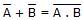# Electronics - Logic Circuit Simplification - Discussion

### Discussion :: Logic Circuit Simplification - General Questions (Q.No.9)

9.

One of DeMorgan's theorems states that. Simply stated, this means that logically there is no difference between:

 [A]. a NAND gate and an AND gate with a bubbled output [B]. a NOR gate and an AND gate with a bubbled output [C]. a NOR gate and a NAND gate with a bubbled output [D]. a NAND gate and an OR gate with a bubbled output

Explanation:

No answer description available for this question.

 Sooryashibu said: (Feb 25, 2014) NAND = NOT of AND.

 Lakshmi said: (Apr 21, 2015) Can you please elaborate?

 Abedelkareem said: (Jul 11, 2015) I think that, the correct answer is D. Because a NAND is equivalent to after equal term and OR with bubbled output is equivalent for the before equal term.

 Scaneu said: (Aug 31, 2016) I think it should be: OR gate with bubble on each input is equivalent to a NAND gate.

 Mohan said: (Jun 11, 2017) Option B is Correct. A NOR gate and a bubbled input of AND gate.

 Madarasi said: (Jun 11, 2017) I think option A is correct. Because, NAND gate output=A.B whole bar=A bar+B bar. The Output of AND gate is A.B = bubbled output=A.B whole bar.

 Affan said: (Mar 17, 2018) Option A is correct. As ( A.B whole bar = Abar + Bbar) is the result of only NAND gate. Therefore option A as both gates are NAND in this option only.

 Sneha said: (Jan 15, 2019) I think Option D is correct. As (A.B whole bar = Abar + Bbar) is the result of only NAND gate and (Abar + Bbar=bubbled OR).